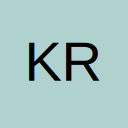# Convert Text to Number within a column

• Hi,

I have a column in which I have the revenue of numerous companies, but they show up in shorthand form "\$491.80M" to "\$2.06B", how would I go about converting these to actual numbers "491,000,000" and "2,060,000,000"?

In the past I have just filtered out the M or B for these values then multiplied the value by 1,000,000 or 1,000,000,000 respectively. But I would like to create a macro for this as it is very time consuming.

i was thinking of a formula along the lines of:

Dim Reng As Range
Dim h As Range
ColNum = Cells.Find(What:="Revenue").Column
LastRow = Cells(Rows.Count, ColNum).End(xlUp).Row
Set Reng = Range(Cells(1, ColNum), Cells(LastRow, ColNum))
For Each h In Reng
If h.Value contains "M" Then
h.Value = "mulitply by 1000000"
End If
Next h

^ I know the above is wrong, but any help would be greatly appreciated!

• Re: Convert Text to Number within a column

Lets assume you have the following in column A
\$491.80M
\$2.06B
The following code will convert the text in Column A and put the real value in Column B. The assumptions are that each revenue entry starts with a "\$" and ends with with "B" or "M"

I hope this helps
Anthony

PS: You could do this without VBA using the following conditional statement within the worksheet

Code
``=IF(RIGHT(RC[-1],1)="M",MID(RC[-1],2,(LEN(RC[-1])-2))*1000000,MID(RC[-1],2,(LEN(RC[-1])-2))*1000000000)``

If Column 1 contains e.g. \$2.06M then this formula would convert it to 2060000. This assumes there only two conditions ->B or M

Just another option

Regards
[SIZE=3]Anthony
[/SIZE]&WCF_AMPERSAND[SIZE=3]
[/SIZE]&WCF_AMPERSAND&WCF_AMPERSAND&WCF_AMPERSAND[SIZE=2]You have your way. I have my way. As for the right way, the correct way, and the only way, it does not exist.[/SIZE]

## Participate now!

Don’t have an account yet? Register yourself now and be a part of our community!